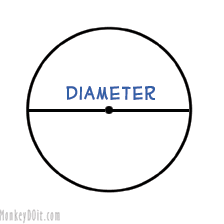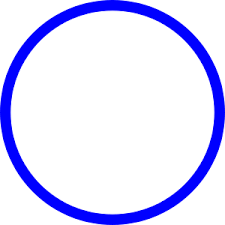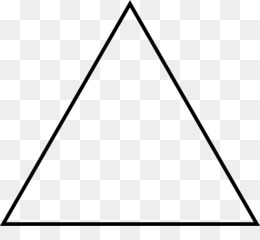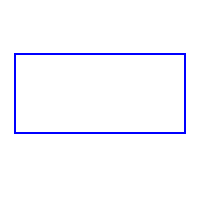# What is the definition of the diameter of the circle. nnWhat is circle? nWhat is triangle? nWhat is rectangle? nWhat is square

Solution:

Diameter of a circle:

The diameter is the length of the line through the center that touches two points on the edge of the circle.Circle:
A circle is a shape consisting of all points in a plane that are a given distance from a given point, the centre; equivalently it is the curve traced out by a point that moves in a plane so that its distance from a given point is constant.Triangle:

A triangle is a polygon with three edges and three vertices. It is one of the basic shapes in geometry. A triangle with vertices A, B, and C is denoted. In Euclidean geometry, any three points, when non-collinear, determine a unique triangle and simultaneously, a unique planeRectangle:

In Euclidean plane geometry, a rectangle is a quadrilateral with four right angles. It can also be defined as an equiangular quadrilateral, since equiangular means that all of its angles are equal. It can also be defined as a parallelogram containing a right angleSquare:

In geometry, a square is a regular quadrilateral, which means that it has four equal sides and four equal angles. It can also be defined as a rectangle in which two adjacent sides have equal length